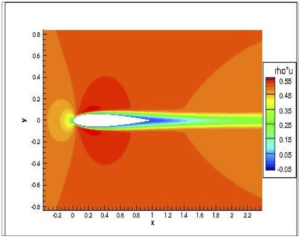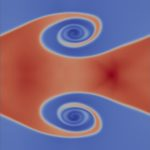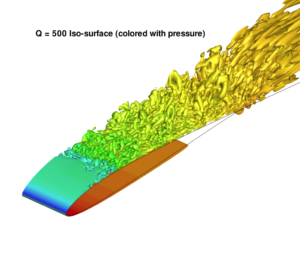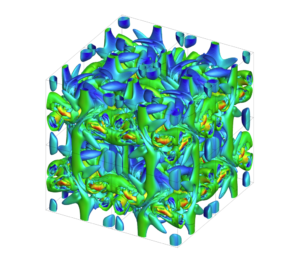## About me

I am a Professor of Applied and Computational Mathematics at the University of Bergen. Most of my research is, one way or another, connected to compressible flows and aerodynamics governed by the Euler and Navier-Stokes equations.

## Numerical analysisSeparated but stable flow around a NACA0012 airfoil.

A mathematical model that aspires to predict future events has to be well-posed. Hence, well-posedness of time-dependent Partial Differential Equations (PDEs) is a recurring theme of my research. For linar PDEs, the energy method is used to derive an a priori bound on the solution and deduce boundary conditions. The analysis is then mimicked for semi-discrete approximations satisfying a Summation-by-parts (SBP) property and with boundary conditions enforced by Simultaneous Approximation Terms (SAT). This leads to stable high-order accurate schemes.

Another key question is the convergence rate of SBP-SAT schemes. The order of accuracy is easily derived for finite difference schemes, but it drops near boundaries for high-order schemes. However, the convergence rate is often higher than the worst case scenario and the question is: What are the weakest conditions for an improved convergence rate? Recent results suggest that energy stable schemes often have optimal convergence rates.

(The work on linear PDEs is not limited to aerodynamics but generally applicable to a large class of linear time-dependent PDEs.)

## Numerical analysis of non-linear conservation laws

The overarching goals are the same as for linear PDEs. However, the mathematical theory is much less developed and well-posedness is largely unknown. This complicates the development of numerical schemes. One way to obtain some a prioriKelvin-Helmholtz roll-ups

bounds is to enforce entropy stability on the scheme. (A kind generalization of the energy stability in the linear case.) My work on such schemes has been aimed towards: extending them to high-order of accuracy; including boundary conditions in the entropy stability framework; enforce both entropy stability as well as additional bounds. The long term goal is to have a scheme that satisfies strong enough bounds to allow convergence to weak solutions.

## Compressible flow models

The inability to prove well-posedness, or even existence of solutions, for the Navier-Stokes equations may well be a consequence of how a compressible fluid is modelled. Hence, I have studied the basic assumptions upon which the Navier-Stokes equations are derived. This has led me to propose a model of compressible viscous flows that is entirely derived in an Eulerian (fixed in space), rather than Lagrangian frame (moving fluid parcel), with diffusion as the main physical principle rather than viscous stresses. Preliminary comparisons indicate that the new model is as accurate as the standard Navier-Stokes model, but many more test cases must be investigated, as well as its mathematical properties.

## High performance computing

To demonstrate the efficacy of the schemes it is necessary to run simulations of realistic problems. Such problems invariably requires  large-scale computing facilities. Hence, the schemes are coded (in F90 and MPI) and run on parallel computers.Turbulence on a 3D wing.Turbulence generated by Taylor-Green Vortex.